• +91 9971497814
• info@interviewmaterial.com

# Coordinate Geometry Ex-7.3 Interview Questions Answers

### Related Subjects

Question 1 :

Find the area of thetriangle whose vertices are:

(i) (2, 3), (-1, 0), (2, -4)

(ii) (-5, -1), (3, -5), (5, 2)

Solution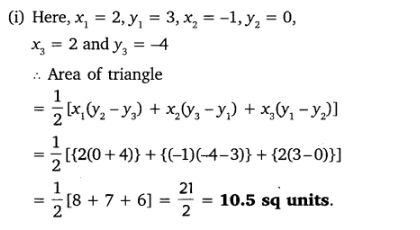Solution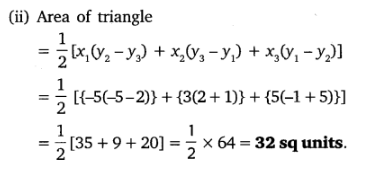Question 2 :

In each of thefollowing find the value of ‘k’, for which the points are collinear.

(i) (7, -2), (5, 1), (3, -k)

(ii) (8, 1), (k, -4), (2, -5)

Solution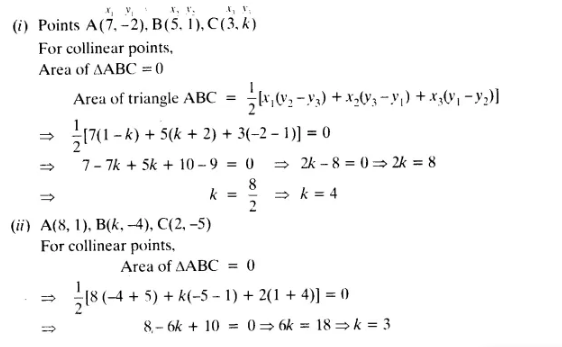Question 3 :

Find the area of thetriangle formed by joining the mid-points of the sides of the triangle whosevertices are (0, -1), (2, 1) and (0, 3). Find the ratio of this area to thearea of the given triangle.

Answer 3 :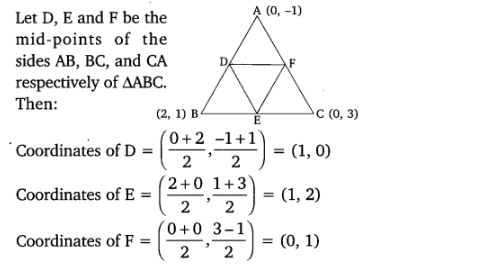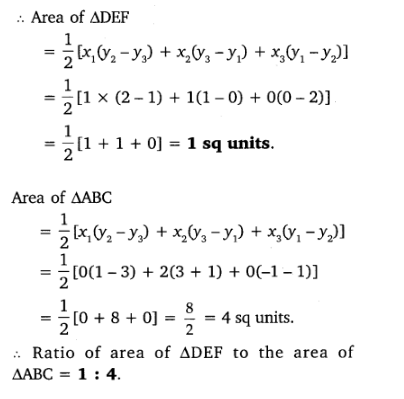Question 4 :

Find the area of thequadrilateral whose vertices, taken in order, are

(-4, -2), (-3, -5),(3, -2) and (2, 3).

Answer 4 :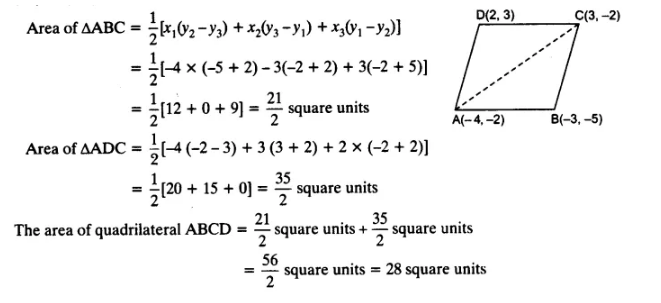Question 5 : You have studied in Class IX that a median ofa triangle divides it into two triangles of equal areas. Verify this result forΔABC whose vertices are A (4, – 6), B (3, – 2) and C (5, 2).

Answer 5 :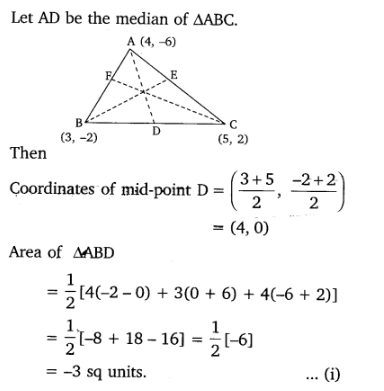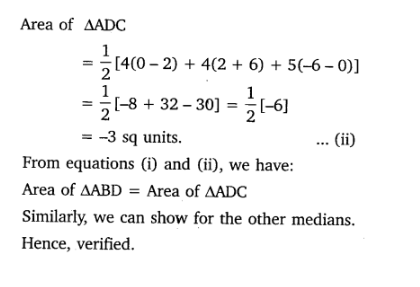Todays Deals### Coordinate Geometry Ex-7.3 Contributorskrishan

Name:
Email:

# Latest News# 9000 interview questions in different categories